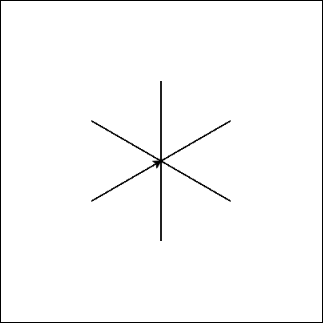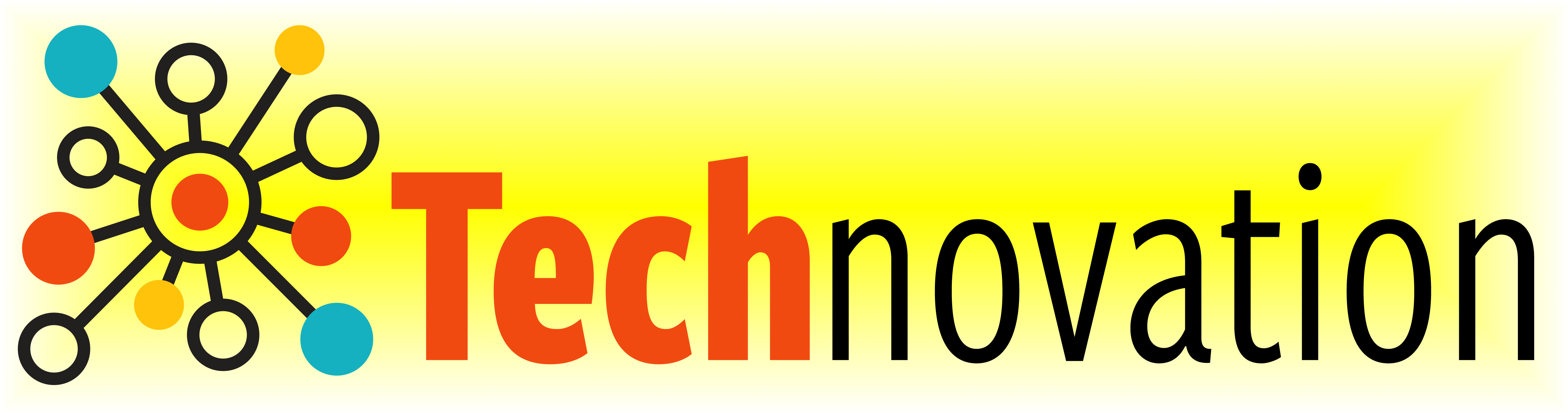# 2.5. Practice Makes Perfect¶

Let’s take another look at drawing a square. How could we re-write it using a loop?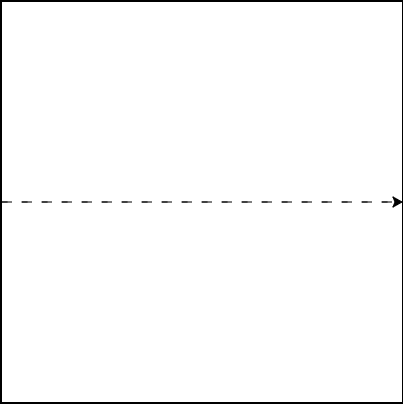Let’s use what we know about loops to draw a dashed line across the entire box!
The code below draws two dashes. See if you can modify it to use a loop!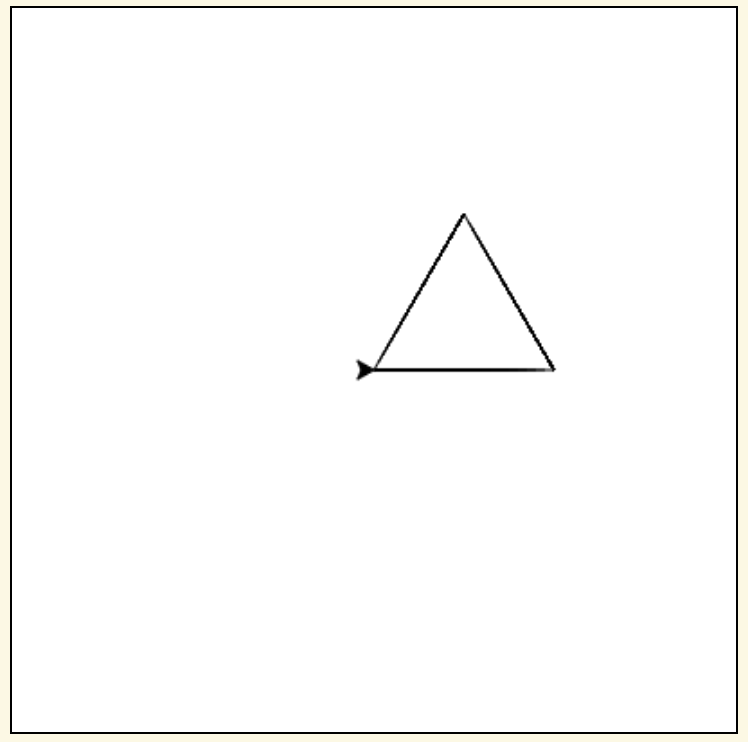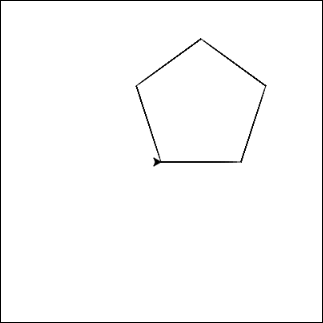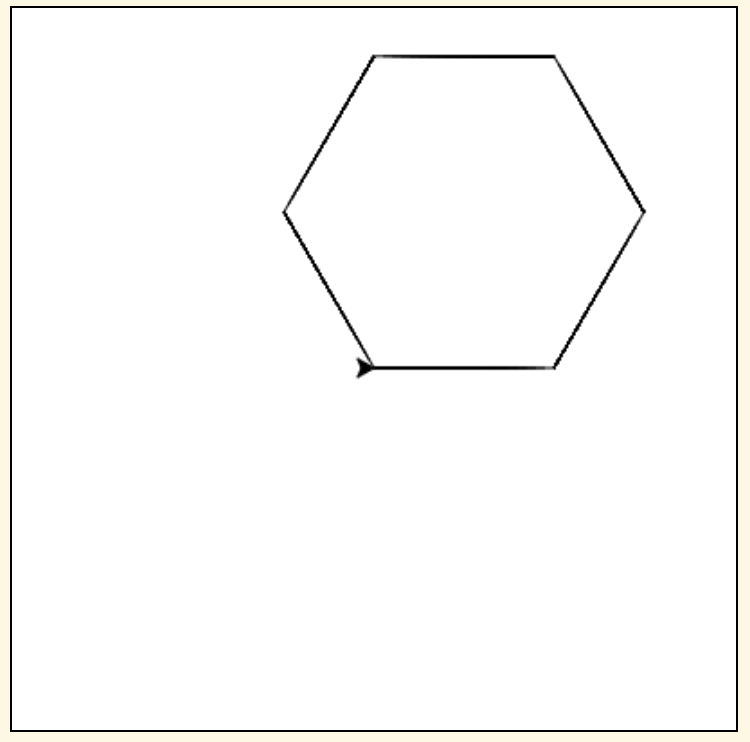Why stop with squares? Shapes with any number of sides can be drawn using a loop!
Start with your code that draws a square. Then, change the number of times the loop repeats and the angle that you turn each time to see if you can draw these shapes.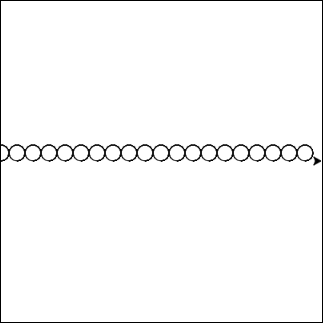Try converting you dashed line into a row of circles! Let’s draw 20 circles, each with a radius of 10.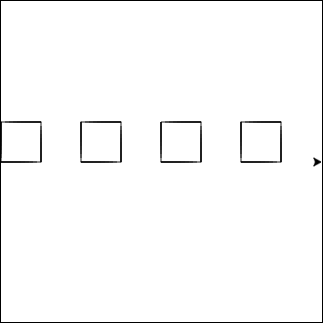Try drawing a row of squares!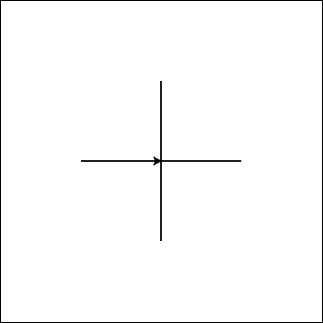Try drawing a plus sign using a loop!
If you’re not sure how to do it with a loop, try writing it out line by line first. Once you’ve got it drawing a plus sign, see if you can put any repeated code in a loop!